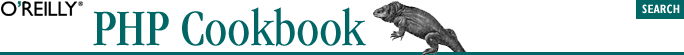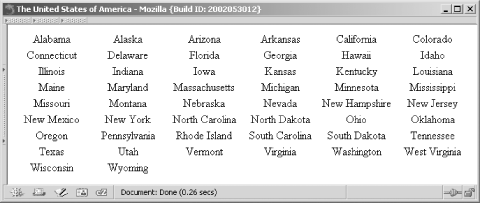home | O'Reilly's CD bookshelfs | FreeBSD | Linux | Cisco | Cisco Exam## 4.27. Program: Printing an Array in a Horizontally Columned HTML Table

The function pc_grid_horizontal( ) , shown in Example 4-8, lets you specify an array and number of columns. It assumes your table width is 100%, but you can alter the \$table_width variable to change this.

#### Example 4-8. pc_grid_horizontal( )

```function pc_grid_horizontal(\$array, \$size) {

// compute <td> width %ages
\$table_width = 100;
\$width = intval(\$table_width / \$size);

// define how our <tr> and <td> tags appear
// sprintf() requires us to use %% to get literal %
\$tr = '<tr align="center">';
\$td = "<td width=\"\$width%%\">%s</td>";

// open table
\$grid = "<table width=\"\$table_width%\">\$tr";

// loop through entries and display in rows of size \$sized
// \$i keeps track of when we need a new table tow
\$i = 0;
foreach (\$array as \$e) {
\$grid .= sprintf(\$td, \$e);
\$i++;

// end of a row
// close it up and open a new one
if (!(\$i % \$size)) {
\$grid .= "</tr>\$tr";
}
}

// pad out remaining cells with blanks
while (\$i % \$size) {
\$grid .= sprintf(\$td, '&nbsp;');
\$i++;
}

\$end_tr_len = strlen(\$tr) * -1;
if (substr(\$grid, \$end_tr_len) != \$tr) {
\$grid .= '</tr>';
} else {
\$grid = substr(\$grid, 0, \$end_tr_len);
}

// close table
\$grid .= '</table>';

return \$grid;
}```

The function begins by calculating the width of each <td> as a percentage of the total table size. Depending on the number of columns and the overall size, the sum of the <td> widths might not equal the <table> width, but this shouldn't effect the displayed HTML in a noticeable fashion. Next, define the <td> and <tr> tags, using printf-style formatting notation. To get the literal % needed for the <td> width percentage, use a double %%.

The meat of the function is the foreach loop through the array in which we append each <td> to the \$grid. If you reach the end of a row, which happens when the total number of elements processed is a multiple of number of elements in a row, you close and then reopen the <tr>.

Once you finish adding all the elements, you need to pad the final row with blank or empty <td> elements. Put a nonbreaking space inside the data cell instead of leaving it empty to make the table renders properly in the browser. Now, make sure there isn't an extra <tr> at the end of grid, which occurs when the number of elements is an exact multiple of the width (in other words, if you didn't need to add padding cells). Finally, you can close the table.

For example, let's print the names of the 50 U.S. states in a six-column table:

```// establish connection to database
\$dbh = DB::connect(\$dsn);
if (DB::isError(\$dbh)) { die (\$dbh->getMessage( )); }

// query the database for the 50 states
\$sql = "SELECT state FROM states";
\$sth = \$dbh->query(\$sql);

// load data into array from database
while (\$row = \$sth->fetchRow(DB_FETCHMODE_ASSOC)) {
\$states[ ] = \$row['state'];
}

// generate the HTML table
\$grid = pc_grid_horizontal(\$states, 6);

// and print it out
print \$grid;```#### Figure 4-1. The United States of America